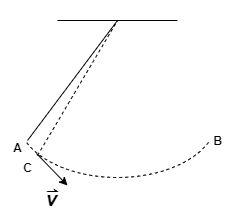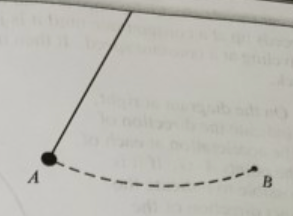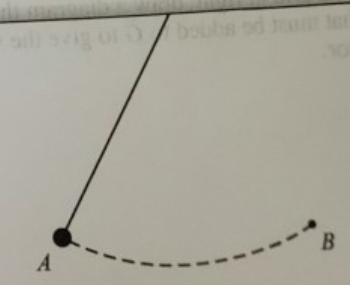# Problem: A pendulum bob swings back and forth. At the instant shown, the bob is at one of the turnaround points, labeled A. The other turnaround point, labeled B, and the bob’s trajectory (dashed) are shown.a. Choose a point slightly after point A, and label it point C. Draw a vector to represent the velocity of the bob at point C.b. Determine the change-in-velocity vector v between points A and C.c. How would you characterize the direction of v as point C is chosen to lie closer and closer to point A?d. Each of the following statements is incorrect. Discuss the flaws in the reasoning.i. “The acceleration at point A is zero. As point C becomes closer and closer to point A, the change-in-velocity vector becomes smaller and smaller. Eventually, it becomes zero.”ii. “At point A, the acceleration makes an angle with the tangent to the trajectory that is greater than 0° but less than 90° because the trajectory is curved and the object is speeding up.”e. On the diagram at right, draw arrows at points A and B to indicate the direction of the acceleration at those points. (Hint: Your answer should be consistent with your answer to parts c and d.) Explain.

###### FREE Expert Solution

a.

The velocity is tangential (perpendicular) to the trajectory.

We'll pick a point close to A and label it C then draw an arrow perpendicular to the radius.The arrow labeledrepresents the velocity of the bob at point C

95% (213 ratings)###### Problem Details

A pendulum bob swings back and forth. At the instant shown, the bob is at one of the turnaround points, labeled A. The other turnaround point, labeled B, and the bob’s trajectory (dashed) are shown.a. Choose a point slightly after point A, and label it point C. Draw a vector to represent the velocity of the bob at point C.

b. Determine the change-in-velocity vector v between points A and C.

c. How would you characterize the direction of v as point C is chosen to lie closer and closer to point A?

d. Each of the following statements is incorrect. Discuss the flaws in the reasoning.
i. “The acceleration at point A is zero. As point C becomes closer and closer to point A, the change-in-velocity vector becomes smaller and smaller. Eventually, it becomes zero.”
ii. “At point A, the acceleration makes an angle with the tangent to the trajectory that is greater than 0° but less than 90° because the trajectory is curved and the object is speeding up.”

e. On the diagram at right, draw arrows at points A and B to indicate the direction of the acceleration at those points. (Hint: Your answer should be consistent with your answer to parts c and d.) Explain.